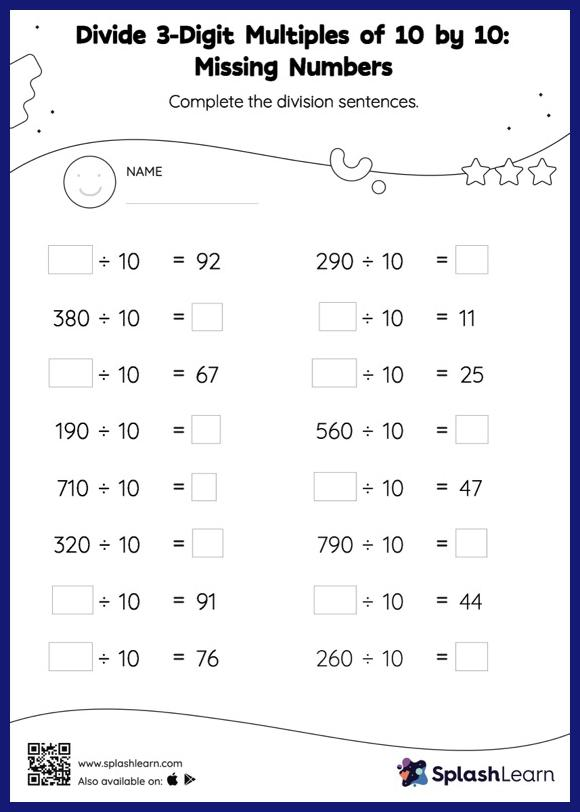# Divide 3-Digit Multiples of 10 by 10: Missing Numbers Worksheet

Home > Divide 3-Digit Multiples of 10 by 10: Missing NumbersDoes your child know how to divide 3-digit multiples of 10 by 10? Invite them to practice this concept here. When dividing by 10 or its multiples, students look at the number of zeros at the end of the dividend or divisor to determine the quotient. They use the same understanding to find the missing number in divide 3-digit multiples of 10 by 10 worksheet. In each problem, the numbers are laid out in the horizontal format. Students should try to use different strategies involving composing and decomposing numbers to solve these problems. This will help them develop flexibility and fluency.